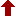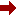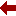#### SLIM21

 Sort Order Chronological Author Title ItemType,Author ItemType,Title Display Format Tabular Publication Bibliography Items / Page 5 10 25

Click the serial number on the left to view the details of the item.
#  AuthorTitleAccn#YearItem Type Claims
1 Hiroshi Isozaki Maxwell equation: Inverse Scattering in Electromagnetis 026588 2018 Book
2 V. Balakrishnan Mathematical physics: With Applications, Problems & Solutions 026231 2017 Book
3 Washek F. Pfeffer Divergence theorem and sets of finite perimeter 024787 2012 Book
4 Matheus Grasselli Numerical mathematics 022370 2008 Book
5 Peter Baxandall Vector calculus 003735 1986 Book
6 T.A. Ramos Lecons sur le calcul vectoriel 005927 1930 Book
 (page:1 / 1) [#6]

1.TitleMaxwell equation: Inverse Scattering in Electromagnetis
Author(s)Hiroshi Isozaki
PublicationNew Jersey, World Scientific Publishing Co. Pvt. Ltd., 2018.
Descriptionxv,283p.
Abstract NoteHow can one determine the physical properties of the medium or the geometrical properties of the domain by observing electromagnetic waves? To answer this fundamental problem in mathematics and physics, this book leads the reader to the frontier of inverse scattering theory for electromagnetism. The first three chapters, written comprehensively, can be used as a textbook for undergraduate students. Beginning with elementary vector calculus, this book provides fundamental results for wave equations and Helmholtz equations, and summarizes the potential theory. It also explains the cohomology theory in an easy and straightforward way, which is an essential part of electromagnetism related to geometry. It then describes the scattering theory for the Maxwell equation by the time-dependent method and also by the stationary method in a concise, but almost self-contained manner. Based on these preliminary results, the book proceeds to the inverse problem for the Maxwell equation. The chapters for the potential theory and elementary cohomology theory are good introduction to graduate students. The results in the last chapter on the inverse scattering for the medium and the determination of Betti numbers are new, and will give a current scope for the inverse spectral problem on non-compact manifolds. It will be useful for young researchers who are interested in this field and trying to find new problems.
ISBN,Price9789813232693 : \$ 118.00(HB)
Classification537.8
Keyword(s)1. COHOMOLOGY 2. MAXWELL EQUATION 3. POTENTIAL THEORY 4. SCATTERING THEORY 5. VECTOR CALCULUS
Item TypeBook

Circulation Data
 Accession# Call# Status Issued To Return Due On Physical Location 026588 537.8/ISO/026588 On Shelf

+Copy Specific Information
2.TitleMathematical physics: With Applications, Problems & Solutions
Author(s)V. Balakrishnan
PublicationNew Delhi, Ane Books Pvt. Ltd, 2017.
Descriptionxxvi, 852p
ISBN,Price9789386761118 : 2495.00(PB)
Classification531.1:51(076)/BAL/026231
Keyword(s)1. ELECTROMAGNETIC THEORY - MATHEMATICAL PHYSICS 2. FLUID MECHANICS - MATHEMATICAL PHYSICS 3. LINEAR RESPONSE THEORY - MATHEMATICAL PHYSICS 4. LINEAR VECTOR SPACES 5. MATHEMATICAL PHYSICS 6. MATHEMATICAL PHYSICS - EXERCISES AND PROBLEMS 7. QUANTUM MECHANICS - MATHEMATICAL PHYSICS 8. QUANTUM OPTICS - MATHEMATICAL PHYSICS 9. RANDOM PROCESS - MATHEMATICAL PHYSICS 10. SCATTERING THEORY - MATHEMATICAL PHYSICS 11. SPECIAL RELATIVITY - MATHEMATICAL PHYSICS 12. VECTOR CALCULUS
Item TypeBook

Circulation Data
 Accession# Call# Status Issued To Return Due On Physical Location 026231 531.1:51(076)/BAL/026231 On Shelf

+Copy Specific Information
3.TitleDivergence theorem and sets of finite perimeter
Author(s)Washek F. Pfeffer
PublicationBoca Raton, CRC Press, 2012.
Descriptionxv, 242p.
Abstract NoteThis book is devoted to a detailed development of the divergence theorem. The framework is that of Lebesgue integration â no generalized Riemann integrals of HenstockâKurzweil variety are involved. In Part I the divergence theorem is established by a combinatorial argument involving dyadic cubes. Only elementary properties of the Lebesgue integral and Hausdorff measures are used. The resulting integration by parts is sufficiently general for many applications. As an example, it is applied to removable singularities of CauchyâRiemann, Laplace, and minimal surface equations. The sets of finite perimeter are introduced in Part II. Both the geometric and analytic points of view are presented. The equivalence of these viewpoints is obtained via the functions of bounded variation. These functions are studied in a self-contained manner with no references to Sobolevâs spaces. The coarea theorem provides a link between the sets of finite perimeter and functions of bounded variation. The general divergence theorem for bounded vector fields is proved in Part III. The proof consists of adapting the combinatorial argument of Part I to sets of finite perimeter. The unbounded vector fields and mean divergence are also discussed. The final chapter contains a characterization of the distributions that are equal to the flux of a continuous vector field.
ISBN,Price9781466507197 : UKP 63.99(HB)
Classification517.43
Keyword(s)1. DIVERGENCE THEOREM 2. LEBESGUE INTEGRAL 3. SETS OF FINITE PERIMETER 4. VECTOR CALCULUS
Item TypeBook

Circulation Data
 Accession# Call# Status Issued To Return Due On Physical Location 024787 517.43/PFE/024787 On Shelf

+Copy Specific Information
4.TitleNumerical mathematics
Author(s)Matheus Grasselli;Dmitry Pelinovsky
PublicationNew Delhi, Narosa Publishing House, 2008.
Descriptionxiv, 668p.
ISBN,Price9788173199790 : Rs. 375.00(PB)
Classification519.6
Keyword(s)1. DIFFERENTIAL CALCULUS 2. EIGENVALUES 3. LINEAR SYSTEMS 4. NUMERICAL MATHEMATICS 5. NUMERICAL METHODS 6. ORTHOGONALITY 7. POLYNOMIAL FUNCTIONS 8. SPECTRAL METHOD 9. VECTOR CALCULUS
Item TypeBook

Circulation Data
 Accession# Call# Status Issued To Return Due On Physical Location 022370 519./GRA/022370 On Shelf

+Copy Specific Information
5.TitleVector calculus
Author(s)Peter Baxandall;Hans Liebeck
PublicationOxford, Clarendon press, 1986.
Description550pp.;Bib. pp. 545
Series(Oxford Applied Mathematics and Computing Science series)
Classification517.43
Keyword(s)1. VECTOR ANALYSIS 2. VECTOR CALCULUS
Item TypeBook

Circulation Data
 Accession# Call# Status Issued To Return Due On Physical Location 003735 517.43/BAX/003735 On Shelf

+Copy Specific Information
6.TitleLecons sur le calcul vectoriel
Author(s)T.A. Ramos
PublicationParis, Librairie Scientifique Albert Blanchard, 1930.
Description119pp.
Classification517.43
Keyword(s)VECTOR CALCULUS
Item TypeBook

Circulation Data
 Accession# Call# Status Issued To Return Due On Physical Location 005927 517.43/RAM/005927 On Shelf

+Copy Specific Information
 (page:1 / 1) [#6]